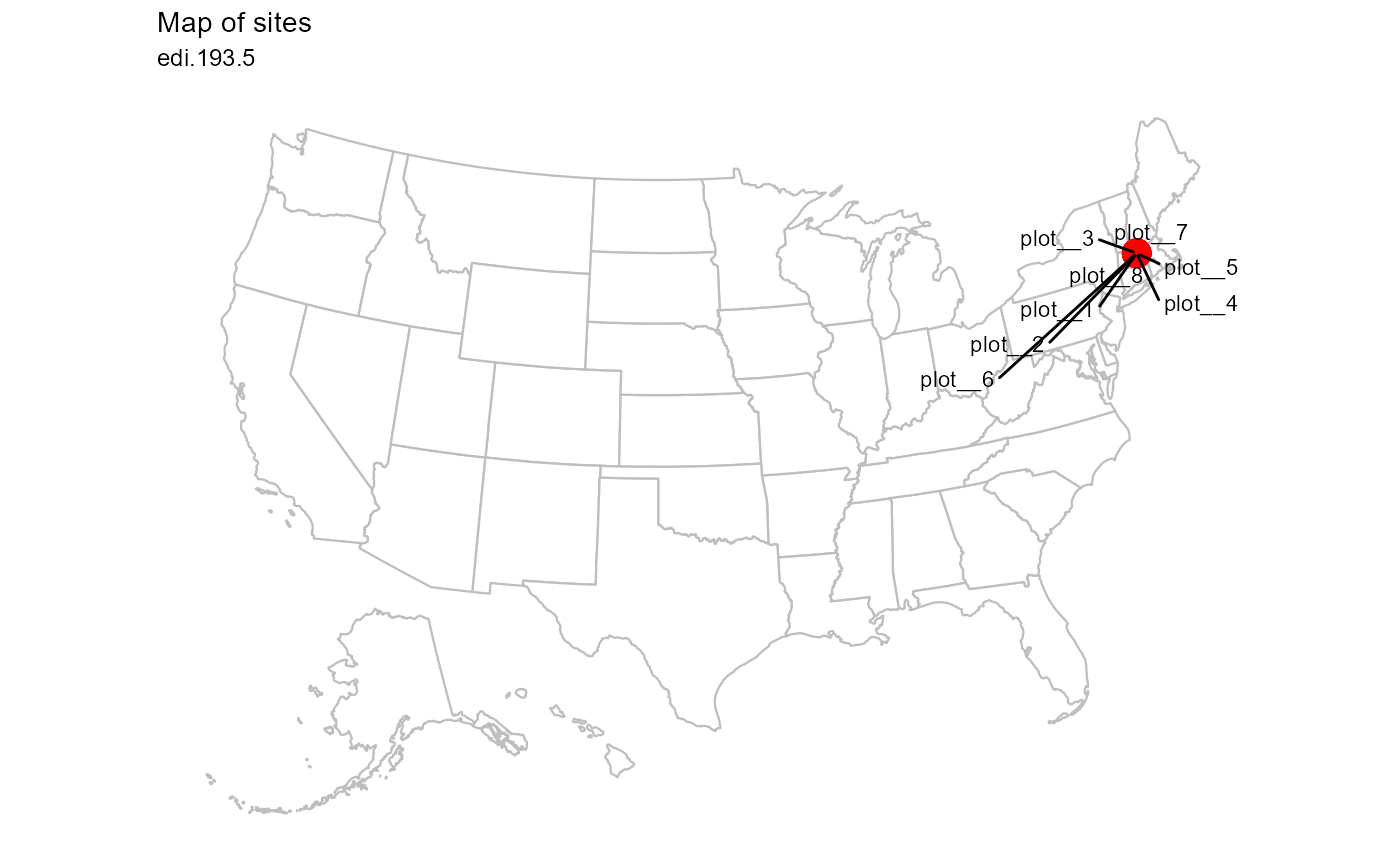Plot sites on US map

plot_sites(data, id = NA_character_, alpha = 1, labels = TRUE)

Arguments

data (list or tbl_df, tbl, data.frame) The dataset object returned by read_data(), a named list of tables containing the observation and taxon tables, or a flat table containing columns of the observation and location tables. (character) Identifier of dataset to be used in plot subtitles. Is automatically assigned when data is a dataset object containing the id field, or is a table containing the package_id column. (numeric) Alpha-transparency scale of data points. Useful when many data points overlap. Allowed values are between 0 and 1, where 1 is 100% opaque. Default is 1. (logical) Argument to show labels of each US state. Default is TRUE.

Value

(gg, ggplot) A gg, ggplot object if assigned to a variable, otherwise a plot to your active graphics device

Details

The data parameter accepts a range of input types but ultimately requires the 14 columns of the combined observation and location tables.

Examples

if (FALSE) { # Read a dataset of interest dataset <- read_data("edi.193.5") # Plot the dataset plot_sites(dataset) # Flatten dataset then plot dataset %>% flatten_data() %>% plot_sites() } # Plot the example dataset plot_sites(ants_L1)
#> Warning: Discarded datum unknown in Proj4 definition